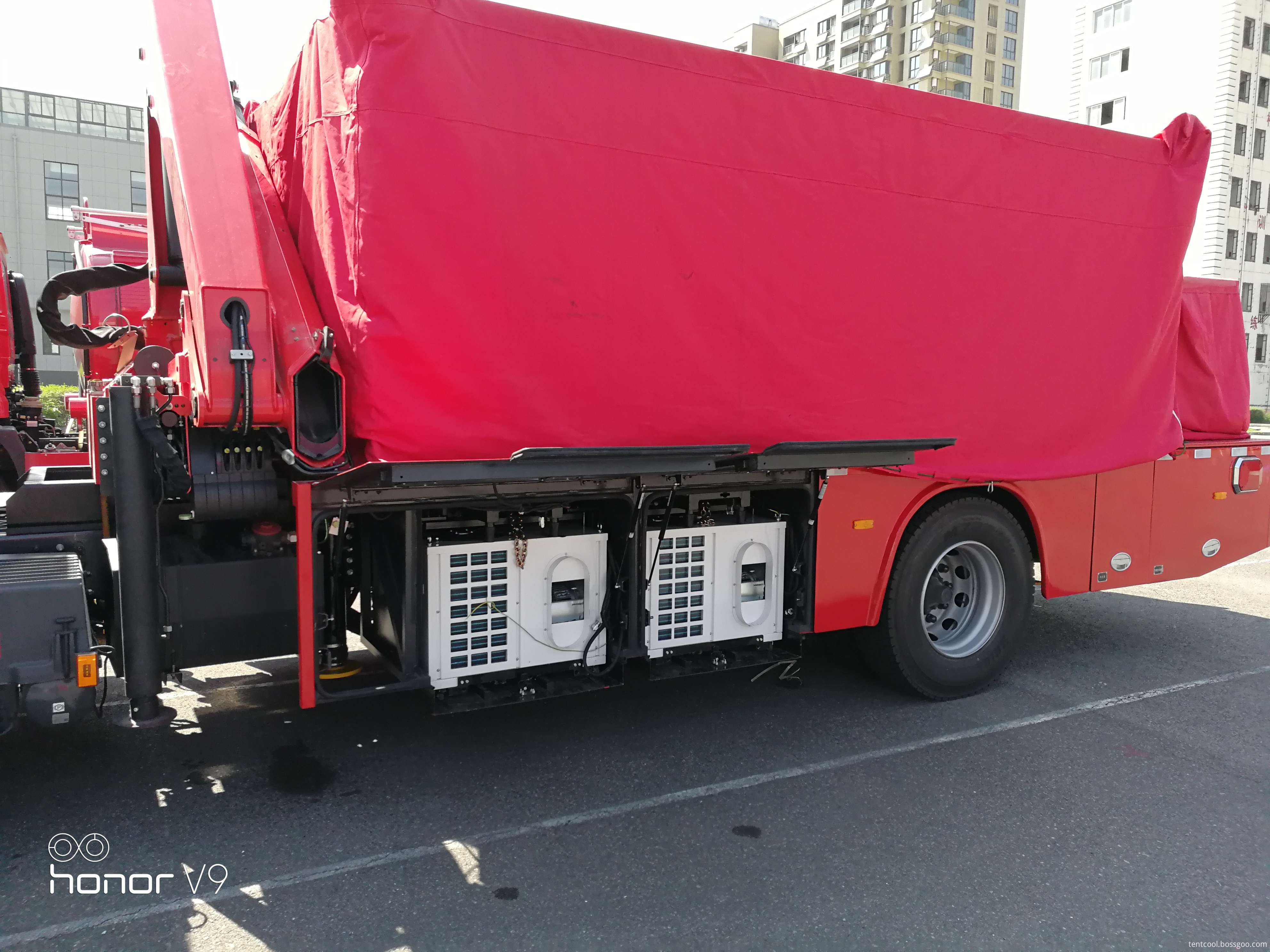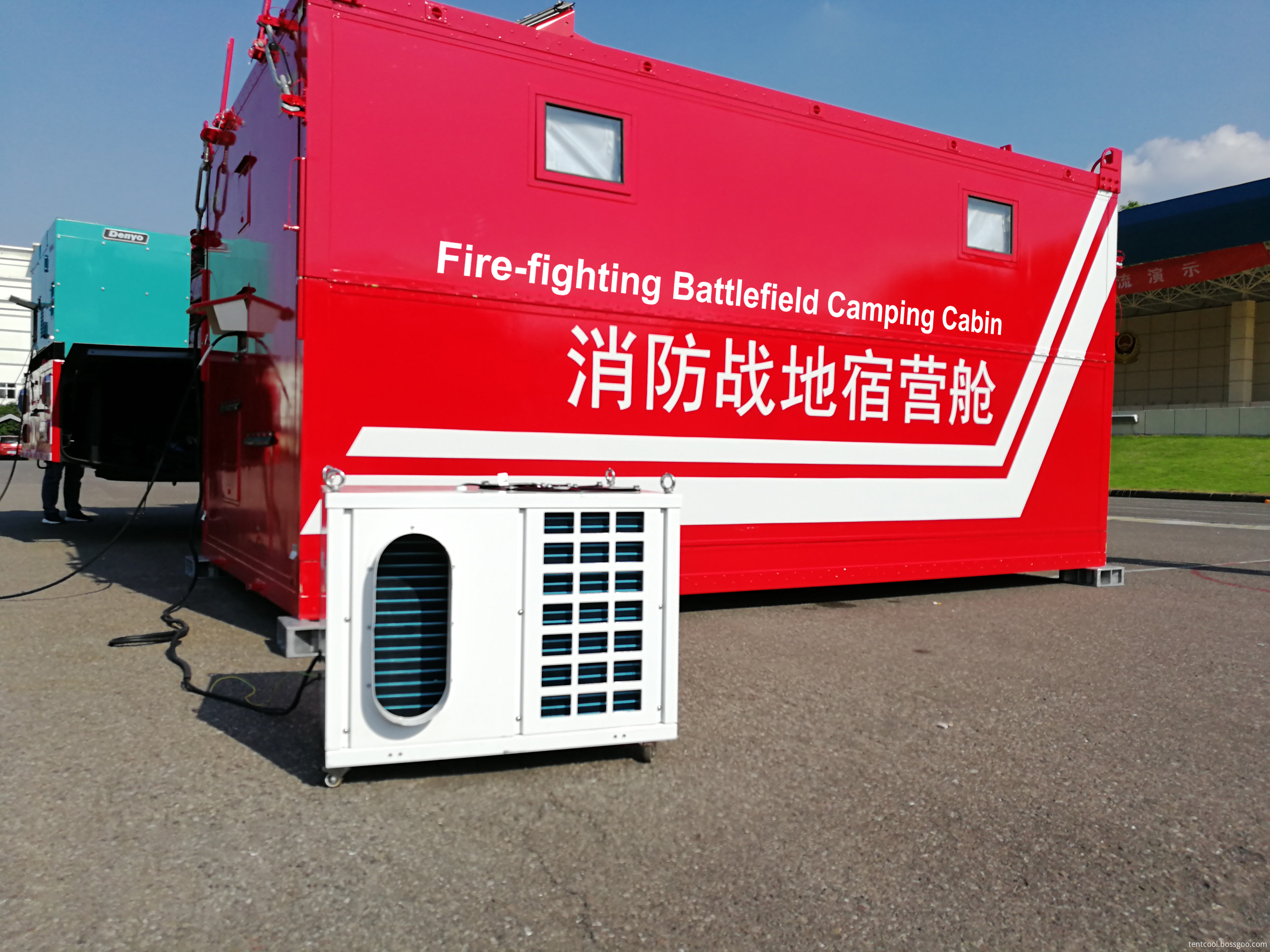There are many documents for designing the crank and rocker mechanism according to the given stroke speed ratio coefficient, but none of the problems of the transmission angle are considered. The size of the transmission angle has a great influence on the transmission performance of the mechanism. In many cases, the crankshaft mechanism has been proposed before its design. How can we meet the requirements? This solves the design problem of the crank-rocker mechanism that satisfies the stroke speed ratio coefficient and also satisfies the transmission angle. The expression of the analytical algorithm under four different conditions is introduced and can be used when designing.

1 basic equation based on transmission angleFigure 1 Crank rocker mechanism minimum transmission angle position

Figure 1 shows a crank rocker mechanism. The lengths of crank AB, link BC, rocker CD, and stand AD are a, b, c, and d, respectively. The acute angle Î³ between the force F acting on the rocker CD by the crank and the force Fn in the rocker direction is the transmission angle. The smaller the Î³, the worse the transmission performance of the mechanism, so there is a limit to the minimum Î³min of the transmission angle. If the angle between the connecting rod and the pendulum is Î´, when Î´ is an acute angle, Î³=Î´; when Î´ is an obtuse angle, Î³=180Â°-Î´. Î´ changes with the change of crank angle Ï†. From Figure 1 can be introduced:(1)

According to formula (1), when Î´=Î´min or Î´=Î´max, the minimum transmission angle Î³min occurs, and the transmission angles Î³â€² and Î³â€²â€² are set here.(2)

Î³â€²=Î´min (when Î´min<90Â°) or Î³â€²=180Â°-Î´min (when Î´min>90Â°)(3)

Î³â€²â€²=Î´max (when Î´max<90Â°) or Î³â€²â€²=180Â°-Î´max (when Î´max>90Â°)
The minimum value among Î³â€² and Î³â€²â€² is the minimum transmission angle Î³min of the mechanism.
Formula (2), (3) can be introduced:
2ad-bc(cosÎ´min-cosÎ´max)=0 (4)

For the crank rocker mechanism shown in Fig. 2 (A and D are on the same side of Câ€²Câ€²â€²), the minimum transmission angle Î³min is at Ï†=0, and Î´min=Î³min (see Reference 2).If Î³min is known, Then Î´min=Î³min is known; if Î´max is given again, equation (4) is the basic equation for a given transmission angle.

2 Basic equations given by the given ratio of travel ratiosFigure 2 Two-level position of crank rocker mechanism

Figure 2 shows the crank rocker mechanism in two extreme positions. The angle between C1D and C2D is the swing angle of the rocker; the AC1 and AC2 lines are the two collinear positions of the crank and the connecting rod; R is the radius of the circumscribed circle caused by the three points C1, C2, and P; h is D, O Distance between two points.
Let âˆ C1C2A=Î±,Î± describe the position of point A in the center of crank rotation. In order to be able to satisfy the continuity condition of the mechanism movement, point A can only beSelect on.
If the stroke speed coefficient is K and the angle between the poles is Î¸, then Î¸=180Â°(k-1)/(k+1).(7)

Equations (5), (6), and (7) are basic equations obtained from the trip speed coefficient.

3 analytic algorithm expression

In Equations (4), (5), (6), and (7), if one of K, Î¨, Î´max, Î´min, and a, b, c, d is known, the other three can be found. Rod length. Here we introduce four different parsing algorithm expressions under known conditions. Due to space limitations, the derivation process is omitted and the results are only listed in the table below.

Analytic expression of table crank rocker

Known conditions to be calculated by the parameter parsing algorithm expression K Î¨ bÎ”max cÎ”mina dK Î¨ aÎ”max cÎ”minb dK Î¨ aÎ”max bÎ”minc d d=CD/sinÎ¸ K Î¨ aÎ”max bÎ”minc c c = dsinÎ¸/DThe analytical type can be directly used to design a crank lifter mechanism with a given speeding coefficient and transmission angle. It subtracts the cumbersome process of inspection and transmission, redesign and failure, and makes the design process simple, accurate, reliable, and can be operated on the machine with high efficiency.

The Battlefield Vehicle Cabin Air Conditioner is originally developed for the fire fighting battlefield sleeping cabins, which provides a comfortable rest or sleep condition for the firemen. It, in fact, can be used for all similar fields like trucks, temporary passageway, or small tents for emergency or military use.

Compared with normal window type air conditioner, it doesn't need to reserve a big hole and should take some inside space.

This kind of sleeping cabin air conditioner supplies the air by duct pipes. No need extra installation. The units are easy to be moved by four wheels. Compact design makes the units can be transported along with the vehicles.Sleeping Cabin Air Conditioner Technical Data (2.5kW/3.5kW):

 Model No. TSAC-025HCWa TSAC-035HCWa Function Cooling&Heating Cooling&Heating Cooling capacity kW/BTU 2.5KW/9000BTU 3.5KW/12000BTU Heating capacity kW/BTU 2.70KW/25000BTU 3.85KW/25000BTU Electric source PH-V-Hz 220V 50Hz / 60Hz 220V 50Hz / 60Hz Rated Cooling Power input W 895W 1250W Rated Heating Power input W 954W 1360W Rated Cooling Current input A 4.5A 5.8A Rated Heating Current input A 4.9A 6.3A Evaporator Fan Type Direct Drive Centrifugal Fan Direct Drive Centrifugal Fan Evaporator Side Air flow volume m3/h 500m3/h 600m3/h Static pressure Pa 100 100 Compressor Type Rotary Rotary Brand GMCC GMCC Refrigerant R22/R410a R22/R410a Condenser Fan Type Direct Drive Axial Fan Direct Drive Axial Fan Condener Side Air Flow Volume m3/h 1200m3/h 1500m3/h Noise dB(A) â‰¤52 â‰¤55 Gross /Net Weight kg 88kg/80kg 93kg/85kg Net Dimension (L x W x H) mm 760*610*620 760*610*620 Packing Dimension (L x W x H) mm 800*650*810 800*650*810

Battlefield Vehicle Cabin Air Conditioner

Sleeping Cabin Air Conditioner, Fire fighting camp cabin air conditioner, fire truck cabin air conditioner, Fire fighting vehicle air conditioner

Taizhou Tentcool Electrical Appliance Co., Ltd. , https://www.tentcool.com

Posted on